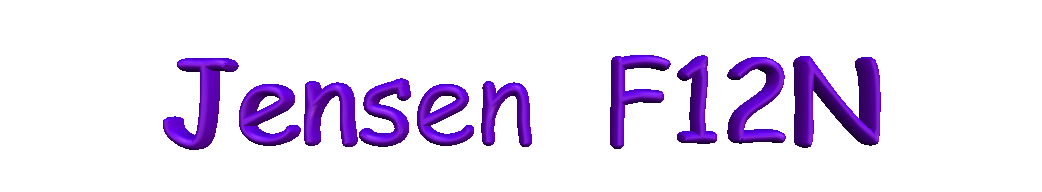During the last couple of years, I've noticed several requests here and on other systems for the Thiele/Small parameters for the venerable Jensen F15 and F12 series. It just so happens that I have a pristine original F12N. Having the equipment to measure such parameters, it was decided to do just that. First, a little info on the unit I have as there have been design changes made over the years.

In this unit, the field coil has a dc resistance of 1003 ohms @ 78 deg. F. The inductance is 2.654 hy @ 60hz and 0.159 hy @ 1 khz. Field coil resistances have been noted to range from as low as 700 ohms to as high as 3500 ohms. If memory serves me well, I've seen higher. I have a 1923 Western Electric FC speaker with a field coil resistance of 3647 ohms.

The voice coil measures 6.3 ohms and the demodulator (humbucking) coil measures 0.2 ohm.

Since this unit was measured with filtered dc applied to the field coil, the demodulator coil serves no purpose other than the cost of about 3% in power transfer from the amplifier to the voice coil. It can be bypassed (shorted) since it is wired in series with the voice coil. This, however, is not recommended unless one has a thorough knowledge of how the speaker is wired as shorting the wrong wires can be disastrous.

There has been much discussion on the actual power supplied to the field coil. The power ranged from 14 watts to about 25 with most recommendations being around 14 to15 watts. This unit was powered with 15w, which is about 123 vdc. The ambient temperature was noted at 78F. After an hour and 14 minutes, the field coil temperature was noted to be 121F and apparently stable. It was then decided to increase the power to 17w (130vdc); eighteen minutes later, the temperature of the field coil was noted to be 126F. Considering the age of the speaker, this level was abandoned.

Tests were made with 10w applied to the field coil and Thiele-Small parameters determined. The biggest difference noted was less than a 1 dB drop in the calculated SPL at 1w1m. It is, therefore, my humble opinion that the power to the field coil be kept around 10-11 watts.

Common sense prevails. Keep in mind these speakers are 50 years old or older. The adhesives holding the voice coil wire to the coil form and the cone to voice coil are considerably aged. There is also the effect of time on the paper cone; it dries and becomes very brittle.

Anyway, here's the Thiele-Small parameters for an 8 ohm Jensen F12N with 15w (dc) applied to the field coil. Included are piccies of normalised amplitude response curves in various size cabinets, closed and vented.

Since there are virtually an infinite number of alignments, internal volumes were kept to about 3 ft^3 or less. Higher low frequency output can be achieved with a vented enclosure of 7 to 10 cubic feet but that size is impractical. The old electric organs typically used such volumes and a lot were essentially open back or covered with a thin panel of about 0.25 inch or thinner. The one exception of which I can think is the Leslie.

As a side note, don't be concerned about the original sound of 50 years ago; it can't be reproduced knowingly. To do that, one would need a time machine to go back to the 40's or 50's and hear a new unit. Suffice it to say that any vintage speaker, especially one with a pristine original cone will sound different than it did back then and in all probability it'll sound different that anything else today. Let's face it, it's all about nostalgia and take it from a 71 year old, that's priceless,.

These next four were determined with 15w to the field coil. (15wFC)

Driver Properties Red curve

Name: F12N 15wFC

Type: Standard one-way driver

Company: JENSEN

No. of Drivers = 1

Fs = 49.77 Hz

Qms = 23.84

Vas = 200.1 liters

Cms = 548.9 µm/N

Mms = 18.63 g

Rms = 0.539 lb/s

Xmax = 2 mm

Xmech = 0.118 in

P-Dia = 10 in

Sd = 0.0507 sq.m

P-Vd = 0.00358 cu.ft

Qes = 0.455

Re = 6.9 ohms

Le = 0.535 mH

Z = 8.28 ohms

BL = 9.4 Tm

Pe = 50 watts

Qts = 0.446

no = 5.184 %

1-W SPL = 99.35 dB

2.83-V SPL = 99.99 dB

-----------------------------------------

Box Properties

Name:

Type: Closed Box

Shape: Prism, square

Vb = 0.783 cu.ft

Qtc = 0.933

QL = 19.94

F3 = 111.4 Hz

Fill = heavy

Driver Properties Orange curve

Optimum closed box alignment (Qtc=0.707)

Name: F12N 15wFC

Type: Standard one-way driver

Company: JENSEN

No. of Drivers = 1

Fs = 49.77 Hz

Qms = 23.84

Vas = 200.1 liters

Cms = 548.9 µm/N

Mms = 18.63 g

Rms = 0.539 lb/s

Xmax = 2 mm

Xmech = 0.118 in

P-Dia = 10 in

Sd = 0.0507 sq.m

P-Vd = 0.00358 cu.ft

Qes = 0.455

Re = 6.9 ohms

Le = 0.535 mH

Z = 8.28 ohms

BL = 9.4 Tm

Pe = 50 watts

Qts = 0.446

no = 5.184 %

1-W SPL = 99.35 dB

2.83-V SPL = 99.99 dB

-----------------------------------------

Box Properties

Name:

Type: Closed Box

Shape: Prism, square

Vb = 1.835 cu.ft

Qtc = 0.707

QL = 17.62

F3 = 96.44 Hz

Fill = heavy

Driver Properties Green curve

Name: F12N 15wFC

Type: Standard one-way driver

Company: JENSEN

No. of Drivers = 1

Fs = 49.77 Hz

Qms = 23.84

Vas = 200.1 liters

Cms = 548.9 µm/N

Mms = 18.63 g

Rms = 0.539 lb/s

Xmax = 2 mm

Xmech = 0.118 in

P-Dia = 10 in

Sd = 0.0507 sq.m

P-Vd = 0.00358 cu.ft

Qes = 0.455

Re = 6.9 ohms

Le = 0.535 mH

Z = 8.28 ohms

BL = 9.4 Tm

Pe = 50 watts

Qts = 0.446

no = 5.184 %

1-W SPL = 99.35 dB

2.83-V SPL = 99.99 dB

-----------------------------------------

Box Properties

Name:

Type: Vented Box

Shape: Prism, square

Vb = 2.065 cu.ft

Fb = 63.57 Hz

QL = 6.772

F3 = 79.94 Hz

Fill = normal

No. of Vents = 1

Vent shape = round

Vent ends = one flush

Dv = 4.558 in

Lv = 0.75 in

Driver Properties Yellow curve

Name: F12N 15wFC

Type: Standard one-way driver

Company: JENSEN

No. of Drivers = 1

Fs = 49.77 Hz

Qms = 23.84

Vas = 200.1 liters

Cms = 548.9 µm/N

Mms = 18.63 g

Rms = 0.539 lb/s

Xmax = 2 mm

Xmech = 0.118 in

P-Dia = 10 in

Sd = 0.0507 sq.m

P-Vd = 0.00358 cu.ft

Qes = 0.455

Re = 6.9 ohms

Le = 0.535 mH

Z = 8.28 ohms

BL = 9.4 Tm

Pe = 50 watts

Qts = 0.446

no = 5.184 %

1-W SPL = 99.35 dB

2.83-V SPL = 99.99 dB

-----------------------------------------

Box Properties

Name:

Type: Vented Box

Shape: Prism, square

Vb = 3.23 cu.ft

Fb = 55.93 Hz

QL = 6.772

F3 = 67.58 Hz

Fill = normal

No. of Vents = 1

Vent shape = round

Vent ends = one flush

Dv = 5.398 in

Lv = 0.75 in

These next two were determined with 10w to the field coil. (10wFC)

Driver Properties Pink curve

Name: F12N 10wFC

Type: Standard one-way driver

Company: JENSEN

No. of Drivers = 1

Fs = 49.63 Hz

Qms = 22.96

Vas = 201.4 liters

Cms = 552.3 µm/N

Mms = 18.62 g

Rms = 0.558 lb/s

Xmax = 2 mm

Xmech = 0.118 in

P-Dia = 10 in

Sd = 0.0507 sq.m

P-Vd = 0.00358 cu.ft

Qes = 0.501

Re = 6.6 ohms

Le = 0.533 mH

Z = 7.92 ohms

BL = 8.746 Tm

Pe = 50 watts

Qts = 0.49

no = 4.698 %

1-W SPL = 98.92 dB

2.83-V SPL = 99.75 dB

-----------------------------------------

Box Properties

Name:

Type: Closed Box

Shape: Prism, square (optimum)

Vb = 2.389 cu.ft

Qtc = 0.707

QL = 16.41

F3 = 87.87 Hz

Fill = heavy

Driver Properties Blue curve

Name: F12N 10wFC

Type: Standard one-way driver

Company: JENSEN

No. of Drivers = 1

Fs = 49.63 Hz

Qms = 22.96

Vas = 201.4 liters

Cms = 552.3 µm/N

Mms = 18.62 g

Rms = 0.558 lb/s

Xmax = 2 mm

Xmech = 0.118 in

P-Dia = 10 in

Sd = 0.0507 sq.m

P-Vd = 0.00358 cu.ft

Qes = 0.501

Re = 6.6 ohms

Le = 0.533 mH

Z = 7.92 ohms

BL = 8.746 Tm

Pe = 50 watts

Qts = 0.49

no = 4.698 %

1-W SPL = 98.92 dB

2.83-V SPL = 99.75 dB

-----------------------------------------

Box Properties

Name:

Type: Vented Box

Shape: Prism, square (optimum)

Vb = 2.661 cu.ft

Fb = 68.85 Hz

QL = 6.642

F3 = 72.83 Hz

Fill = normal

No. of Vents = 1

Vent shape = round

Vent ends = one flush

Dv = 6.59 in

Lv = 0.75 in

In the attached piccy, the trace colors correspond to the colors listed after Driver Properties

The piccy has been enlarged in PhotoShop to facilitate reading the traces.

The vents have been normalised for a tunnel depth of 0.75 inch and are consistent with maximum power tuning.

Another consideration worthy of mention; this is NOT a bass guitar speaker despite it's original use in an electric organ. Those old organ amps delivered about 15 watts and organ tones are not percussive. The transient of a percussive signal fed through a 100 watt or arc welder type amplifier will destroy this unit in short order.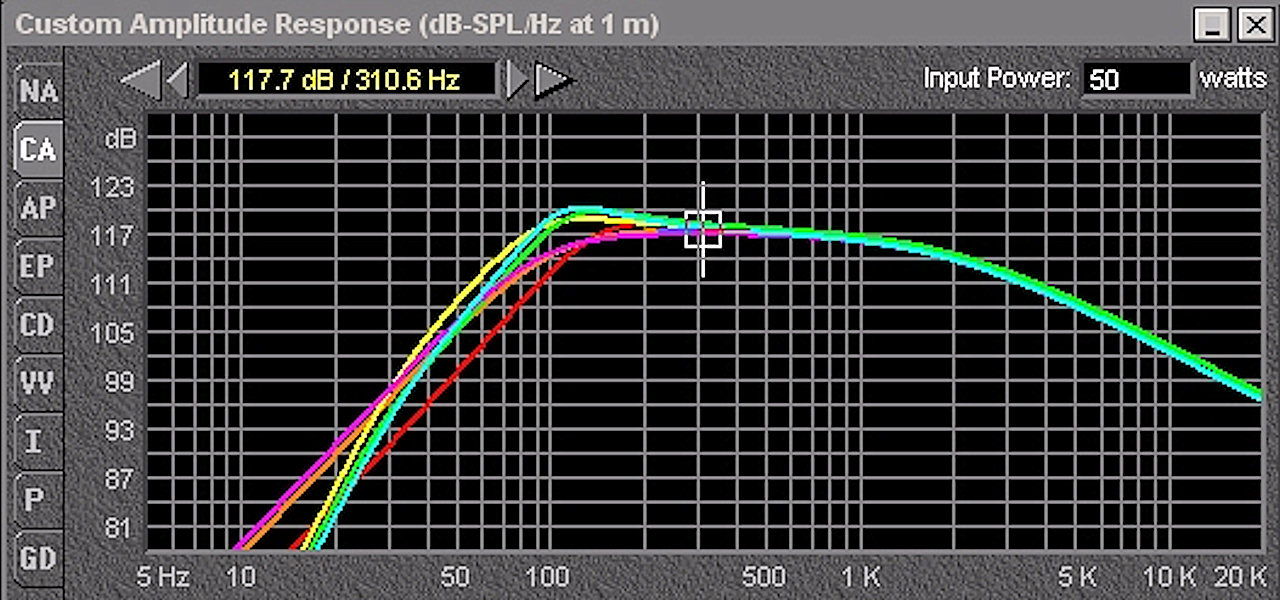Original diaphragm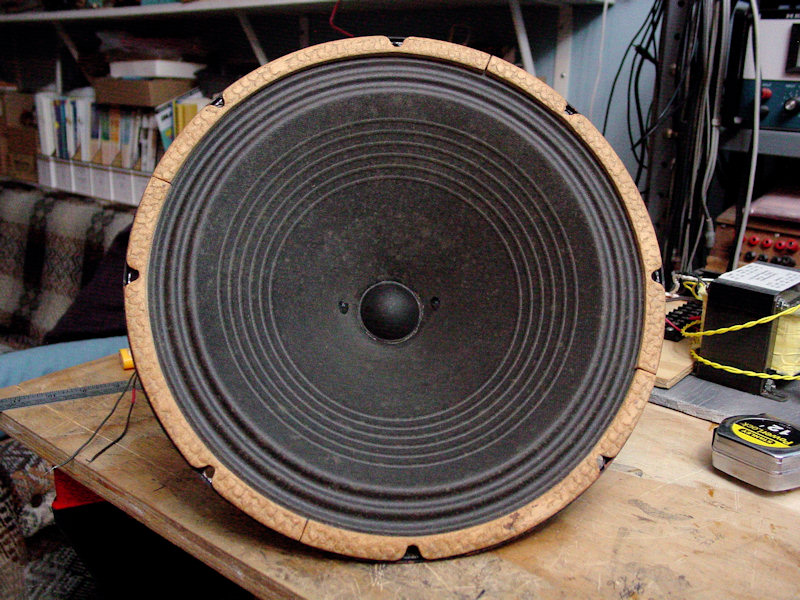Taken outside for diffused lighting - no weird shadows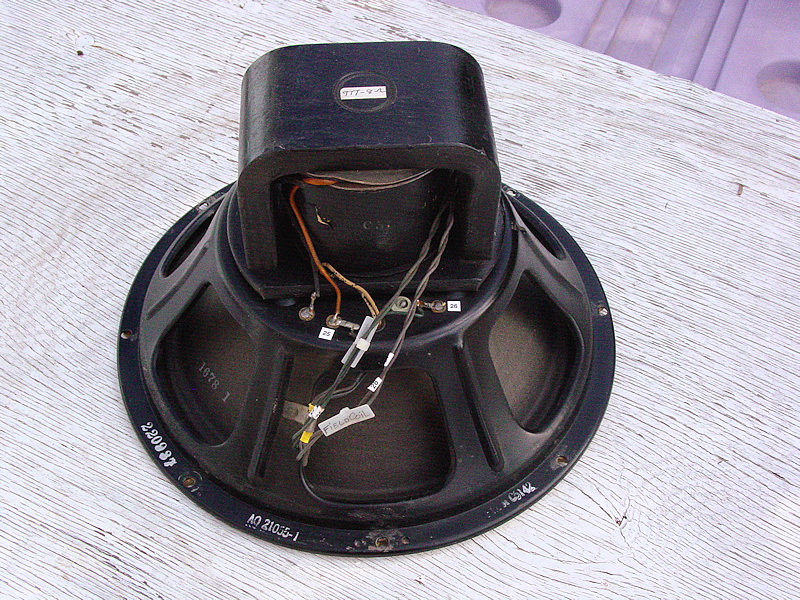The phenolic spider.  If viewed laying flat, it would resemble the critter, hence the name.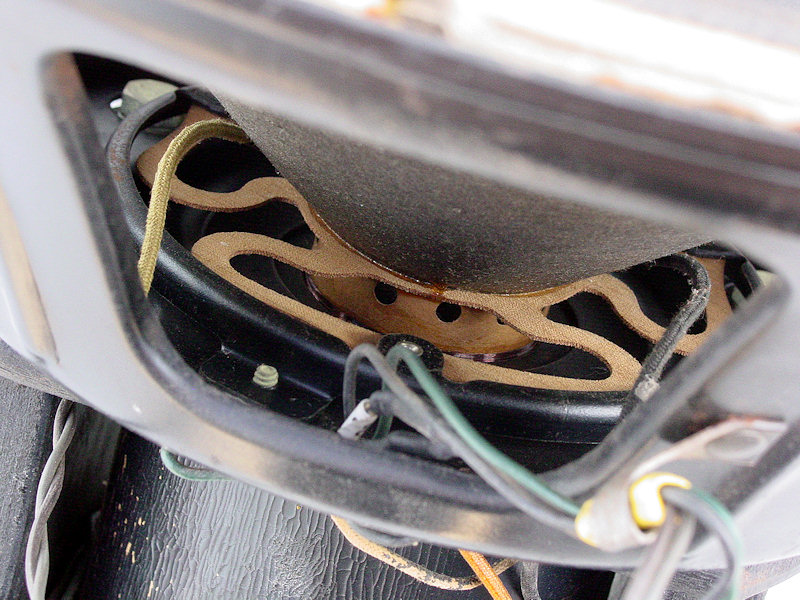F12N vs C12N

Back to the loudspeaker main page